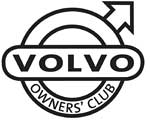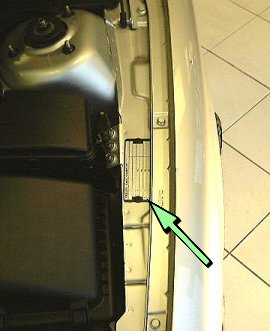# Volvo S80 VIN Plates

1800  |  200  |  300  |  400  |  700  |  850 - 900  |  40/50  |  60  |  70  |  80  |  90  |  XC90

This information will help you to understand the information on the VIN plate of your Volvo car.

Click on the model series number above

Example VIN Number:

YV1TS32G54F123456

YV1 T S 32 G 5 4 F 123456
Manufacturer Code Vehicle Type Doors Engine Type Emission Gearbox Model Year Factory Code Chassis Number

Manufacturer Code
YV1 = cars YV2 = trucks YV3 = buses YV4 = multipurpose 4V1 = trucks 4V2 = 4V3 =
4V4 = trucks 4V5 = trucks 4V6 = 4VL = 4VM = 4VZ = MHA = PT. Central Sole Agency

Vehicle Type
K = S80 BIFUEL T = S80

Doors
A = 4DRS ARMOURED H = AWD (USA)(CDN S = 4DRS U = BI-FUEL LPG V = BI-FUEL CNG

Engine Type
32 = B5244SG 33 = B5244SG2 43 = B5204T3 48 = B5204T4 49 = B5204T5 52 = B5254T4 53 = B5234T3 57 = B5234T7 58 = B5244T3 59 = B5254T2
61 = B5244S 65 = B5244S2 72 = D5252T MSA 15.8 74 = D5244T2 79 = D5244T 90 = B6284T 91 = B6294T 92 = B6294S2 94 = B6294S 97 = B6304S3

Emissions
B = KOD: EM X3 ARABIEN C = KOD: EM L3 D = KOD: EM L6 G = KOD: EM X1 H = KOD: EM X2 (USA)(CDN) K = KOD: EM L4 L = KOD: EM L7 N = KOD: EM T1 P = KOD: EM T2 Q = KOD: EM T3
R = KOD: EM E1 T = KOD: EM T4 V = KOD: EM Z1 (USA)(CDN) W = KOD: EM Z4 X = KOD: EM Z2 Y = KOD: EM Z3 (USA)(CDN) Z = KOD: EM X6 (USA)(CDN) 0 = KOD: EM H1 3 = KOD: EM F1 9 = KOD: EM F2

Gearbox
1 = M66 manual gearbox 2 = M56, M65 manual gearbox 5 = 4T65EV, 4T65EV-GT automatic gearbox 6 = AW50-42 automatic gearbox 9 = AW55-50SN

Model Year
3 = 2003. CH 297000- 4 = 2004. CH 335000-

Model Year From 1980
A = 1980 B = 1981 C = 1982 D = 1983 E = 1984 F = 1985 G = 1986 H = 1987 J = 1988 K = 1989 L = 1990 M = 1991
N = 1992 P = 1993 R = 1994 S = 1995 T = 1996 V = 1997 W = 1998 X = 1999 Y = 2000 1 = 2001 2 = 2002 3 = 2003
4 = 2004 5 = 2005 6 = 2006 7 = 2007 8 = 2008 9 = 2009 A = 2010 (code restarted) B = 2011 C = 2012 D = 2013 E = 2014 ---

Factory Code
0 = Sweden, Kalmar Plant 1 = Sweden, Torslanda Plant VCT 21(Volvo Torslandaverken) (Gothenburg) 2 = Belgium, Ghent Plant VCG 22 3 = Canada, Hatdfax Plant 4 = Italy, - Bertone models 240 5 = Malaysia, 6 = Australia,
7 = Indonesia, A = Sweden, Uddevalla Plant (Volvo Cars/TWR (Tom Walkinshaw Racing)) B = Italy, - Bertone Chongq 31 D = Italy, - Bertone models 780 E = Singapore, F = The Netherlands, Born Plant (NEDCAR) J = Sweden, Uddevalla Plant VCU 38 (Volvo Cars/ Pininfarina Sverige AB)

Chassis/VIN Plate
On the nearside wing facing up on a metal plate held by two black rivets. Later models it has been located on the Right B pillar just under the rear door hinge in a black sticker format.